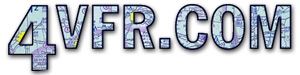Articles | Index

Measures
2010-03-18As pilots we are, generally unaware and/or uneducated, as to the debt that aviation owes to those scientists and mathematicians who preceded the Wright brothers. The mathematics of aviation begins with the prehistoric use of our hands which gave us the span as in wingspan.

Consider the beginning the numbering system. The Babylonians, over 6000 years ago, added the use of symbols, `zero', and place value to make mathematics possible. From the Babylonians we acquired the Base 60 numerical system used in telling time. This base sixty is also part of our navigational system. Ever notice how easy it is to do fractions in Base 60.

It is interesting to note that the sum-of-the-digits for every 45-degrees all around the compass rose is the same. This is true no matter where we start on the rose.
360 = 9;
270 = 9; 090 = 9; 135 = 9

The Egyptians, not much later, devised a way of dividing the year into twelve months even including the leap year. They followed up with land measuring systems not unlike those used in modern map making.

The Greeks developed axioms into theorems and proofs. They used abstractions to illustrate the reality of the world. Such abstractions pilots now call sectionals, charts, or maps. The Greek, Thales, demonstrated five propositions which have become part of geometry or earth measurement.

&middot; A circle is bisected by its diameter. Whenever we draw a course line we are bisecting a circle.
&middot; Equilateral triangles have equal sides and angles. Every time we make a 45 entry to an airport, decide when to turn downwind and determine the `key' point to turn base we are applying our knowledge of equilateral triangles.
&middot; A diagonal through parallel lines give equal opposite angles
A knowledge applied every time we enter on a 45 and enter downwind.
&middot; Triangles having one side equal as well as two angles equal are congruent.

Euclid also collected all the math ideas he could such as all right angles are equal, a straight line can be drawn from one point to another point, and the sum of the angles in a triangle equal 180 degrees. Archimedes was a mathematician as well as a creative engineer. He used a screw shape in tube to move water. The movement of a propeller through the air is such a screw. Early propellers were called air screws. His use of pulleys and levers have applications in aircraft control systems. Pythagoras with his followers discovered the roundness of the earth, the numerical relationships of frequencies, and the Pythagorean Theorem whose hypotenuse we fly during climbs and descents.

WWII navigation with sextant, chronometer, almanac, all of which made possible finding a line of position using the celestial method of Marcq St.-Hilaaire. Radio navigation uses this method of a line of position with intersecting lines for a given position on the line. Only a timing device is required be it a watch or DME.

Comment